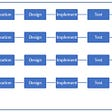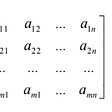# Dimensions

First of all, what the heck is a matrix? Well, a matrix is a “rectangular array of numbers”. In simpler terms, a matrix is a grid where each square holds a value. You might be familiar with matrices in programming (also known as an “array of arrays”). In Java, for example, to create a matrix, you might type this: `int[][] mat = new int`. This would initialize a "2 by 2" matrix. Here is an example of a "2 by 2" matrix in math:

Above is a “matrix of numbers”. Why did I call it “2 by 2”? It has 2 rows and 2 columns. So, for example, this matrix would be a “3 by 2” or just 3x2:

Additionally, the symbol commonly used to represent matrix dimensions is the all-real-numbers symbol: ℝ. The dimensions of the previous example could be written as ℝ^3x2.

# Indexing

Another very common operation needed to be performed on matrices is “indexing”. This is where you can get one value from a matrix. So, if I call the `3x2` matrix above A, and I wanted to index the first element (1), I would say A₁₁. The reason I do this is because matrix indexing occurs by a subscript where the first number corresponds to the row and the second number corresponds the column of the element in the matrix you want to get. Another example: let's suppose I want to index the 6 in the matrix above. It is in the 3rd row and 2nd column, so to index it I would say A₃₂ (Assuming my matrix was called A).

# Vectors

A “vector” is just a matrix but with one column only. You could say it is an `n x 1` matrix because it can have as many rows (n) but only one column. When indexing a vector, you only need one number in the subscript and that corresponds to the row of the number you are indexing. So, if we have this vector

then, Y₁=1, Y₂=2, and Y₃=3.

Just a quick note: all the indexing I have written on is what is called “1-indexing” because the first value in the matrix is referred to with a 1. If you are familiar with programming, then you will most likely be familiar with zero-indexing, where the first value in an array (or matrix) is the 0th element. 1-indexed vectors/matrices are the most common. Another note: matrices and vectors are often named using CAPITOL lettering by convention.

# Simple Operations

Now we will start to perform operations on matrices. For each simple operator (add, subtract, multiply, divide) there are two different matrix operations associated. The two matrix operations are known as “scalar” and “element-wise”. The scalar operator takes a number and performs one operation with each element in the matrix. For example,

This works the same way for subtraction, multiplication, and division using scalar values. With element wise operations, you take two matrices of the SAME dimensions and perform one operation with each corresponding element in each matrix. For example:

Here is an example where you can’t perform the element-wise operation because the two matrices do NOT have the same dimensions:

# Matrix-Vector Multiplication

When multiplying a matrix by a vector, you take each row of the matrix and multiply each element of that row with the corresponding element in the vector and then add them. You do that for each row in the matrix and you end up with a vector with the number of rows as the original matrix. This is known as the “dot product” and is represented with this symbol: “⨂” (or with no symbol like 2x means to multiply 2 with x). It will make more sense once you see this example:

To perform the dot product, we first take the first row of the matrix, [1,3], and multiply each element in it with the corresponding element in the vector like this:

Now you add the values up, 1+15=16. This becomes the first value in the resulting matrix.

You now perform these same steps with the rest of the rows in the matrix. For the sake of brevity, I will put the final operations in the matrix:

And that final matrix is the answer. It’s not difficult to understand, just tedious to execute. This is why NO ONE does this by hand, we use computers to do this for us. In python, using the numpy library, you can just say this to perform that entire dot product: `numpy.dot(matrixA, matrixB)`.

Additionally, because the operations for dot product are so specific, you cannot perform them with just two randomly dimensioned matrices, they have to be specific as well. If the matrix has dimensions ℝᴹˣⁿ(m rows, n columns), then the vector must have the dimensions ℝⁿˣ¹(n dimensional vector). The answer would then be a vector with the dimensions ℝᴹˣ¹.

# Matrix-Matrix Multiplication

To multiply a matrix by another matrix we need to do the “dot product” of the rows and columns … what does that mean? Well, in the previous section, we took a matrix and a vector, and for each row in the matrix, we found the dot product of that row with the vector and ended up with a vector as the result. For multiplying matrices, you do that EXACT same thing, but repeat it for each column in the second matrix acting as individual vectors.

This will make more sense in an example. To work out the answer for the 1st row and 1st column of the resulting matrix in this problem, I would find the dot product of the 1st row of the first matrix and the 1st column of the second matrix like so:

To work out the answer for the 2nd row and 1st column of the resulting matrix, I would find the dot product of the 2nd row of the first matrix and the 1st column of the second like so:

We can do the same thing for the 1st row and the 2nd column:

And for the 2nd row and 2nd column:

And finally, we get:

# The Why

Why is this important to know?

Well, to be honest if you aren’t doing something related to mathematics or computer science (machine learning) I would struggle to give you a good reason that you need to know it. But, for machine learning, it is EXTREMELY useful.

When modeling the layers of a neural network in a program on a computer, each layer can be represented by a vector and the weights as a matrix. Then, when it comes time to forward propagate, the next layer of the network is calculated by the dot product of the previous layer (the vector) and the weights. There are actually many cloud computing services that have computers you can access that are specially designed to be able to perform matrix operations quickly which greatly improves the training process for a network.

This is all I got for this one! Please feel free to email me with any questions 👍📬

--

--

--

## More from Danny Denenberg

Love podcasts or audiobooks? Learn on the go with our new app.

## A brief reminder for technical writing## The Daily PPILL #2 — Processes/Systems## Efficient ways to call .env## Introducing Joseki: the open-source product to improve cloud infrastructure security## Agile development process for my Upgrad Assignment## No-Code Technology: Is it the Future of Blockchain?## What I’ve learned 4 months at Beam## Study notes: An example of Dynamic system imitation by Neural network## Why studying Calculus is important for ML and where to start## Matrix & Matrix Operations | Numerical Linear Algebra | Part 1## Mathematics for Deep Learning (Part 2)